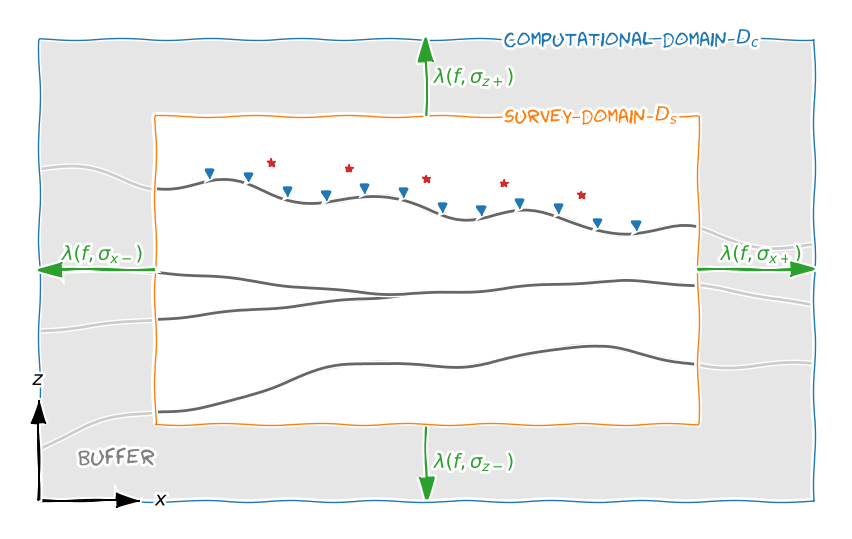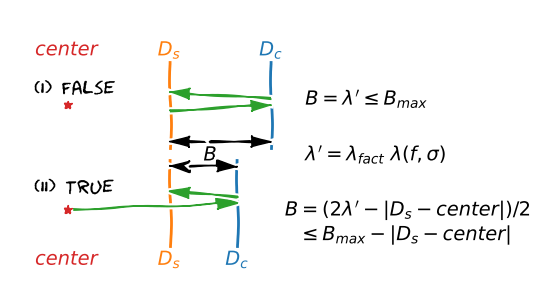# construct_mesh#

emg3d.meshes.construct_mesh(frequency, properties, center, domain=None, vector=None, seasurface=None, **kwargs)[source]#

Return a TensorMesh for given parameters.

Designing an appropriate grid is the most time-consuming part of any 3D modelling:

• Cell sizes should be small enough to represent changes in the model as well as to minimize interpolation errors in the fields.

• The computational domain has to be big enough to avoid effects from the boundary condition.

• The total number of cells should be small to speed up computation.

These are in itself contradictory requirements, and they additionally depend all on the subsurface properties, the frequency under consideration, and the survey type.

This function is a helper routine to construct an appropriate grid. However, there is no guarantee that it is the best or even a good grid. The constructed grid is frequency- and property-dependent. Some details are explained in other functions:

• The minimum cell width $$\Delta_\text{min}$$ is a function of frequency, properties, min_width_pps, and min_width_limits, see Equation (34).

• The skin depth $$\delta$$ is a function of frequency and properties, see Equation (32).

• The wavelength $$\lambda$$ is a function of frequency and properties, see Equation (33).

The relation of the survey domain, computational domain, and buffer zone is shown in Figure 17 for a x-z-section; the y-direction behaves the same as the x-direction (the figures are only visible in the web version of the API docs on https://emg3d.emsig.xyz).Fig. 17 Relation between survey domain (Ds, domain), computational domain (Dc), and buffer zone. The survey domain should contain all sources (red stars) and receivers (blue triangles) as well as any important feature that should be represented in the data. The buffer zone is calculated as a function of wavelength with the provided property in the given direction.#

The buffer zone around the survey domain is by default one wavelength. This means that the signal has to travel two wavelengths to get from the end of the survey domain to the end of the computational domain and back. This approach is quite conservative and on the safe side. You can reduce the buffer thickness if you know what you are doing. There are three parameters which influence the thickness of the buffer for a given frequency: properties, which is used to calculate the skin depth and the wavelength, lambda_factor (default is 1) which sets how many times the wavelength is the thickness of the buffer (relative factor), and max_buffer, which is an absolute maximum for the buffer thickness. A graphical illustration is given in Figure 18.Fig. 18 The thickness of the buffer zone (B) for (I) lambda_from_center=False (default) and for (II) lambda_from_center=True. The lambda_factor ($$\lambda_{fact}$$) is a simple scaling factor for the wavelength $$\lambda$$. The max_buffer is an absolute limitation.#
Parameters
frequencyfloat

Frequency (Hz) to calculate skin depth; both the minimum cell width and the extent of the buffer zone, and therefore of the computational domain, are a function of skin depth.

properties{float, array_like}

Properties to calculate the skin depths, which in turn are used to calculate the minimum cell width (usually at source location) and the extent of the buffer around the survey domain. The properties can be either resistivities, conductivities, or the logarithm (natural or base 10) thereof. By default it assumes resistivities, but it can be changed with the parameter mapping.

Five formats are recognized for properties: it can either be a float or a list of 2, 3, 4, or 7 floats. Depending on the format these properties are used to calculate the following parameters:

• p: min_width and buffer in all directions;

• [p1, p2]:

• p1 : min_width,

• p2 : buffer in all directions;

• [p1, p2, p3]:

• p1 : min_width,

• p2 : buffer in negative z-direction,

• p3 : buffer in all other directions;

• [p1, p2, p3, p4]:

• p1 : min_width,

• p2 : buffer in horizontal directions,

• p3; p4 : buffer in negative; positive z-direction.

• [p1, p2, p3, p4, p5, p6, p7]:

• p1 : min_width,

• p2; p3 : buffer in negative; positive x-direction,

• p4; p5 : buffer in negative; positive y-direction,

• p6; p7 : buffer in negative; positive z-direction.

centerarray_like

Center coordinates (x, y, z). The mesh is centered around this point, which means that here is the smallest cell. Usually this is the source location. Note that from v1.7.0 the default will change: until then, the center is assumed to be at the edge; from v1.7.0 onwards, it is assumed to be at the cell center. It can be changed via the parameter center_on_edge.

domain{tuple, list, dict, None}, optional

Contains the survey-domain limits. This domain should include all source and receiver positions as well as any important feature of the model. Format: ([xmin, xmax], [ymin, ymax], [zmin, zmax]) or {'x': [xmin, xmax], 'y': [ymin, ymax], 'z': [zmin, zmax]}.

It can be None, or individual lists can be None (e.g., (None, None, [zmin, zmax])), in which case you have to provide either the corresponding distance or a vector, from which the domain is then calculated. If only one list is provided it is applied to all dimensions.

distance{tuple, list, dict, None}, optional

An alternative to domain: Instead of defining the domain in absolute values, they are defined here as distance from the center. Format: ([xl, xr], [yl, yr], [zd, zu]) or {'x': [xl, xr], 'y': [yl, yr], 'z': [zd, zu]}. From this the domain is given as ([cx-xl, cx+xr], [cy-yl, cy+yr], [cz-zd, cz+zu]), where center=(cx, cy, cz).

vector{tuple, ndarray, dict, None}, optional

Contains vectors of mesh-edges that should be used. Format: (xvector, yvector, zvector) or {'x': xvector, 'y': yvector, 'z': zvector}.

If also domain or distance is defined, the following happens:

• There must be at least two cells within the domain, otherwise vector is set to None.

• Where the vector is outside the domain, it will be trimmed to it.

• Where the vector is smaller than the domain, it will be extended following the normal rules. The last cell-width in each direction will be taken as starting cell width, together with the domain stretching factor.

It can be None, or individual ndarrays can be None (e.g., (xvector, yvector, None)), in which case you have to provide a domain or distance. If only one ndarray is provided it is applied to all dimensions.

center_on_edge{tuple, bool, dict, None}, optional

Contains booleans defining if center is at the edge or at the cell center. Format: None, bool, (xbool, ybool, zbool) or {'x': xbool, 'y': ybool, 'z': zbool}.

Only relevant if no vector is provided in given direction.

seasurfacefloat, default: None

Air-sea interface, has to be above the center. This has only to be set in the marine case, when the mesh in z-direction is sought for (and the seasurface is not contained in vector). If set, it will try to ensure that at the sea surface is an actual boundary.

If the seasurface lies higher than the survey domain it will increase the survey domain to include the seasurface.

If the seasurface is too close to the center it will most likely fail to find a good grid. It will still create the grid, raising a warning that the seasurface is not at an actual boundary.

stretching{tuple, list, dict}, default: [1.0, 1.5]

Maximum stretching factors in the form of [max Ds, max Dc]: the first value is the maximum stretching for the survey domain (default is 1.0), the second value is the maximum stretching for the buffer zone (default is 1.5). If a list is provided the same is used for all three dimension. Alternatively a tuple of three lists can be provided, (x, y, z) or {'x': x, 'y': y, 'z': z}. Note that the first value has no influence on dimensions where a vector is provided.

min_width_limits{float, list, tuple, dict, None}, default: None

Passed through to cell_width as limits. A tuple of three or a dict with x;y;z can be provided for direction dependent values. Note that this value has no influence on dimensions where a vector is provided.

min_width_pps{float, tuple, dict}, default: 3.0

Passed through to cell_width as pps. A tuple of three or a dict with x;y;z can be provided for direction dependent values. Note that this value has no influence on dimensions where a vector is provided.

lambda_factorfloat, default: 1.0

The buffer is taken as one wavelength from the survey domain. This can be regarded as quite conservative (but safe). The parameter lambda_factor can be used to reduce (or increase) this factor.

max_bufferfloat, default: 100_000

Maximum thickness of the buffer zone around survey domain. If lambda_from_center=True, this is the maximum distance from the center to the end of the computational domain.

lambda_from_centerbool, default: False

Flag how to compute the extent of the computational mesh as a function of wavelength:

• False (default): The distance from the edge of the survey domain to the edge of the computational domain is one wavelength.

• True: The distance from the center to the edge of the computational domain and back to the end of the survey domain is two wavelengths.

mapping{str, Map}, default: ‘Resistivity’

Defines what type the input property_{x;y;z}-values correspond to. By default, they represent resistivities (Ohm.m). The implemented mappings are:

• 'Resistivity'; ρ (Ω m);

• 'Conductivity'; σ (S/m);

• 'LgResistivity'; log_10(ρ);

• 'LgConductivity'; log_10(σ);

• 'LnResistivity'; log_e(ρ);

• 'LnConductivity'; log_e(σ).

cell_numbersarray_like, optional

List of possible numbers of cells. See good_mg_cell_nr. Default is good_mg_cell_nr(1024, 5, 3), which corresponds to numbers 16, 24, 32, 40, 48, 64, 80, 96, 128, 160, 192, 256, 320, 384, 512, 640, 768, 1024.

verbint, default: 0

If 1 verbose, if 0 silent. The info is added either way to the returned mesh as mesh.construct_mesh_info.

Returns
gridTensorMesh

Resulting mesh, a emg3d.meshes.TensorMesh instance.